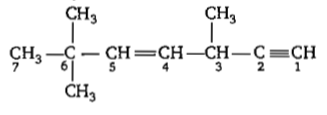Which of the following species contains three bond pairs and one lone pair around the central atom?

(a) H2O                            (b) BF3

(c) ${\mathrm{NH}}_{2}^{-}$                            (d) PCl3

Concept Questions :-

V.S.E.P.R
High Yielding Test Series + Question Bank - NEET 2020

Difficulty Level:

The pair of species with the same bond order is:

(a) ${\mathrm{O}}_{2}^{2-}$, B2

(b) ${\mathrm{O}}_{2}^{+}$, NO-

(c) NO, CO

(d) N2, O2

Concept Questions :-

M.O.T
High Yielding Test Series + Question Bank - NEET 2020

Difficulty Level:

Considering the state of hybridisation of carbon atoms, find out the molecule among the following which is linear.

(a) HC≡CH

(b) CH2 = CH—CH2—C = CH

(c) CH3 — CH2 — CH2 — CH3

(d) CH3—CH = CH—CH3

High Yielding Test Series + Question Bank - NEET 2020

Difficulty Level:

The correct order of increasing bond length of

C-H, C-O, C-C and C=C is

(a) C-C<C=C<C-O<C-H

(b) C-O<C-H<C-C<C=C

(c) C-H<C-O<C-C<C=C

(d) C-H<C=C<C-O<C-C

Concept Questions :-

Covalent Bonding
High Yielding Test Series + Question Bank - NEET 2020

Difficulty Level:

Which of the following compounds has the lowest melting point?

(a) CaBr2

(b) CaI2

(c) CaF2

(d) CaCl2

Concept Questions :-

Lattice/Hydration Energy
High Yielding Test Series + Question Bank - NEET 2020

Difficulty Level:

Which of the following has the minimum bond length?

(a) O2-

(b) O22-

(c) O2

(d) O2+

Concept Questions :-

M.O.T
High Yielding Test Series + Question Bank - NEET 2020

Difficulty Level:

In which of the following pairs of molecules/ions, the central atoms have sp2 hybridisation?

Concept Questions :-

Hybridisation
High Yielding Test Series + Question Bank - NEET 2020

Difficulty Level:

The tendency of BF3, BCl3, and BBr3 to behave as Lewis acid decreases in the sequence

(a) BCl3 > BF3 > BBr3

(b) BBr3 > BCI3 > BF3

(c) BBr3 > BF3 > BCl3

(d) BF3 > BCl3 > BBr3

Concept Questions :-

Covalent Bonding
High Yielding Test Series + Question Bank - NEET 2020

Difficulty Level:

The state of hybridisation of C2, C3, C5 and C6 of the hydrocarbon,is in the following sequence
(a) sp, sp3, sp2 and sp3
(b) sp3, sp2, sp2 and sp
(c) sp, sp2, sp2 and sp3
(d) sp, sp2 sp3 and sp2

Concept Questions :-

Hybridisation
High Yielding Test Series + Question Bank - NEET 2020

Difficulty Level:

In which of the following molecules/ions BF3, ${\mathrm{NO}}_{2}^{-}$, ${\mathrm{NH}}_{2}^{-}$ and H2O, the central atom is sp2 hybridised?
(a) ${\mathrm{NO}}_{2}^{-}$ and ${\mathrm{NH}}_{2}^{-}$
(b) ${\mathrm{NH}}_{2}^{-}$ and H2O
(c) ${\mathrm{NO}}_{2}^{-}$ and H2O
(d) BF3, and ${\mathrm{NO}}_{2}^{-}$

Concept Questions :-

Hybridisation